# Geometry & Trigonometry: College Precalculus Lesson Plans Chapter Exam

Exam Instructions:

Choose your answers to the questions and click 'Next' to see the next set of questions. You can skip questions if you would like and come back to them later with the yellow "Go To First Skipped Question" button. When you have completed the practice exam, a green submit button will appear. Click it to see your results. Good luck!

### Page 1

#### Question 1 1. The lines a and b are parallel lines of length 5 and 2. The lines d and e are parallel to one another, and both are perpendicular to a and b. What is the length of c?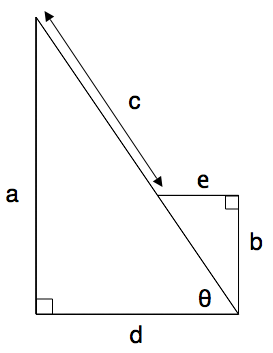#### Question 2 2. What is x?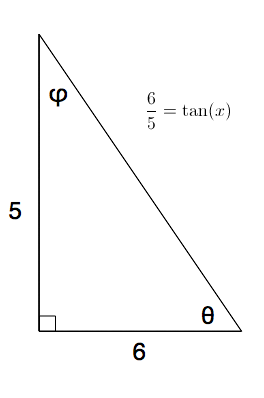#### Question 5 5. What is the area of the right triangle shown below?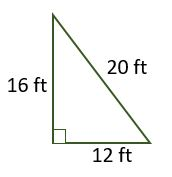### Page 2

#### Question 6 6. Jerry wants to buy a TV online, but the ad for the TV he wants does not say what the screen size is. He knows that TV screens are measured diagonally. The ad states the height and width of the screen, so he uses the Pythagorean Theorem to determine what the screen size is. What is the screen size of the TV that Jerry wants to buy?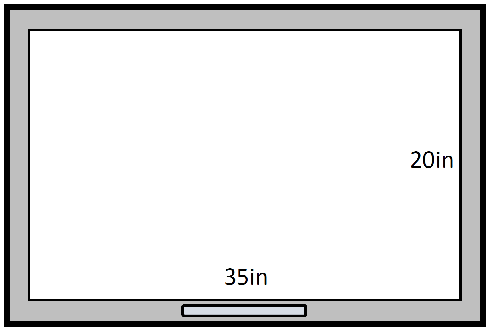#### Question 8 8. What is the area of the annulus/doughnut shown below?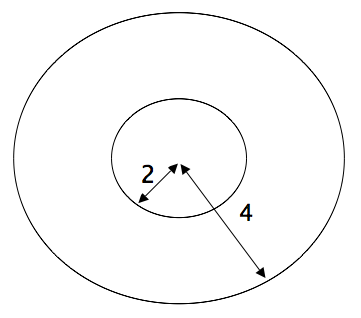#### Question 9 9. Which of the following is true?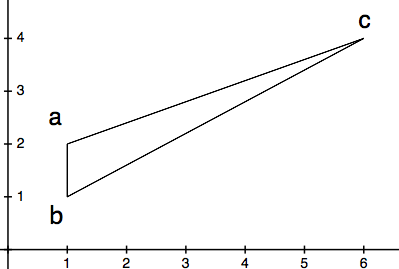#### Question 10 10. What is z in terms of x?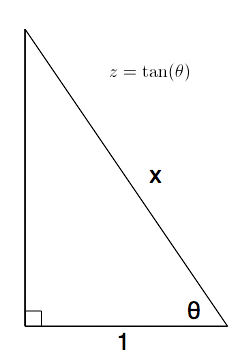### Page 3

#### Question 12 12. What is x?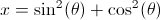#### Question 13 13. What is x?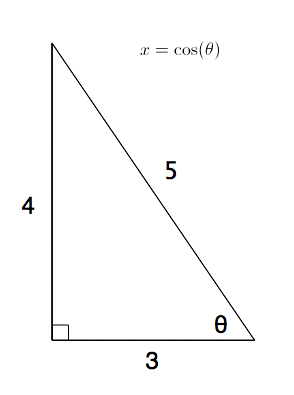#### Question 14 14. What is z in terms of x and y?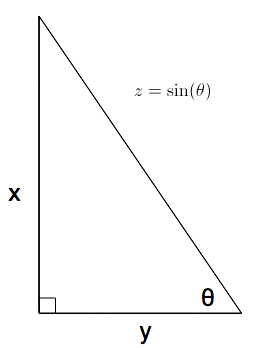#### Question 15 15. What is x?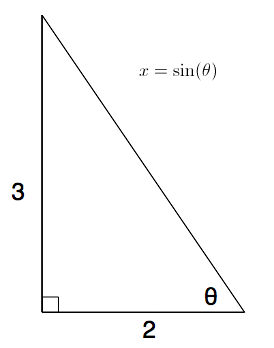### Page 4

#### Question 16 16. What is x?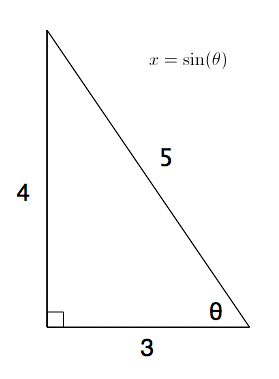#### Question 18 18. What is x?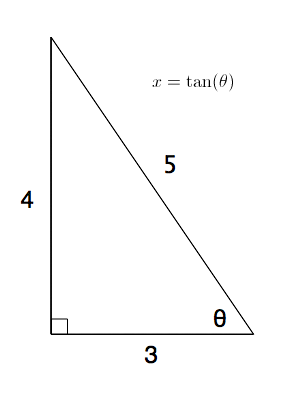### Page 5

#### Question 21 21. Find the shortest distance between a and b by drawing a line connecting them and using the Pythagorean Theorem.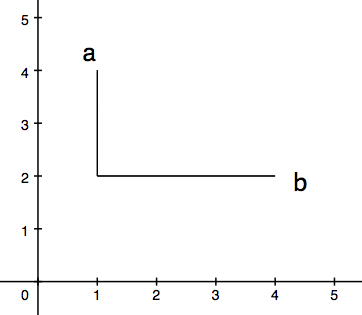#### Question 23 23. What is the area of the diamond/kite shown below?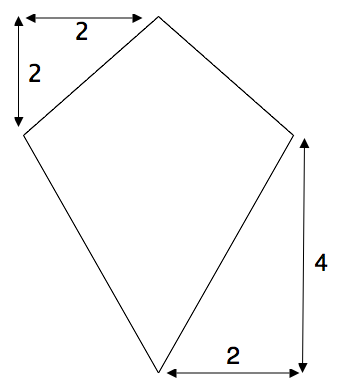#### Geometry & Trigonometry: College Precalculus Lesson Plans Chapter Exam Instructions

Choose your answers to the questions and click 'Next' to see the next set of questions. You can skip questions if you would like and come back to them later with the yellow "Go To First Skipped Question" button. When you have completed the practice exam, a green submit button will appear. Click it to see your results. Good luck!

Support